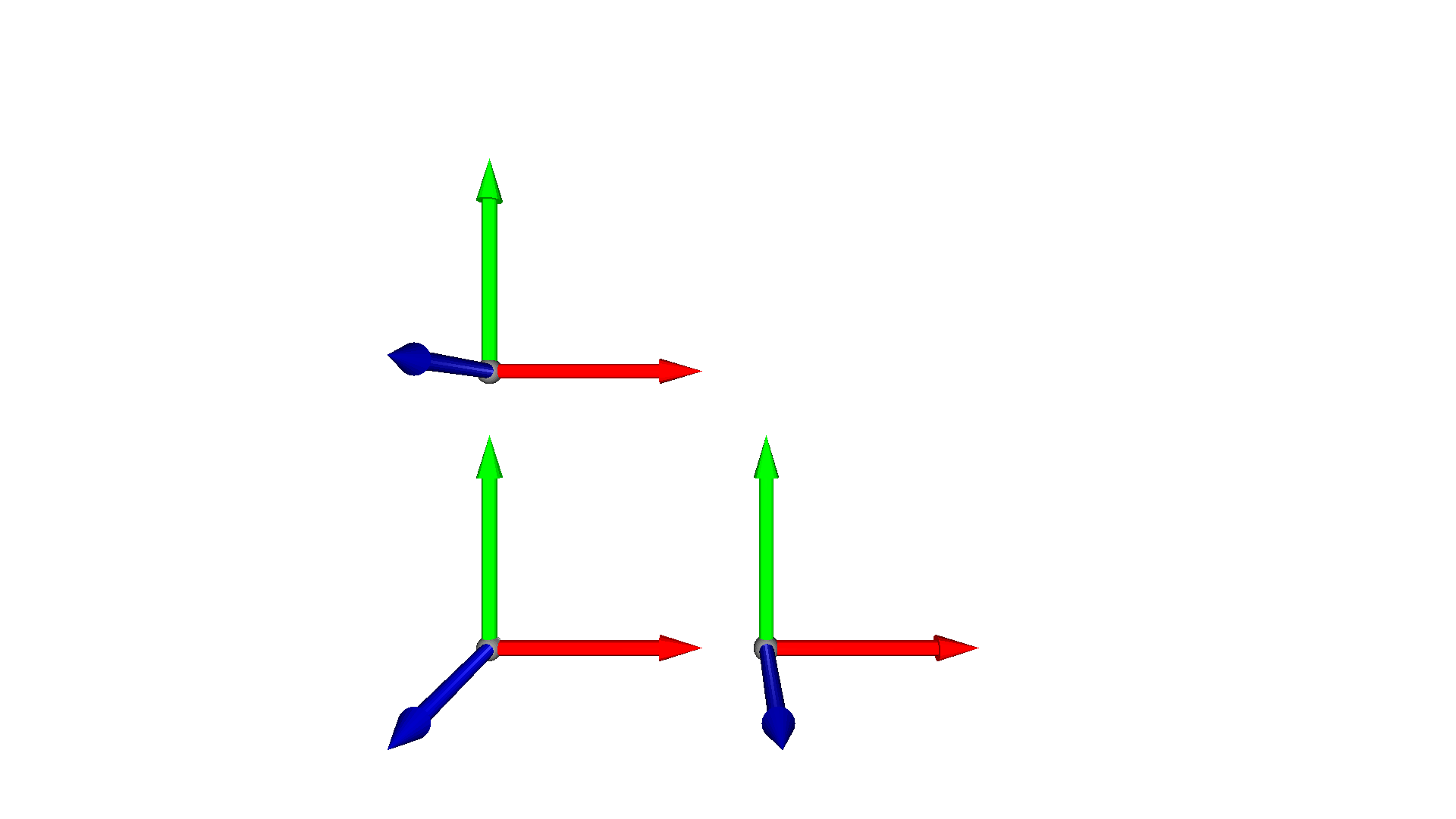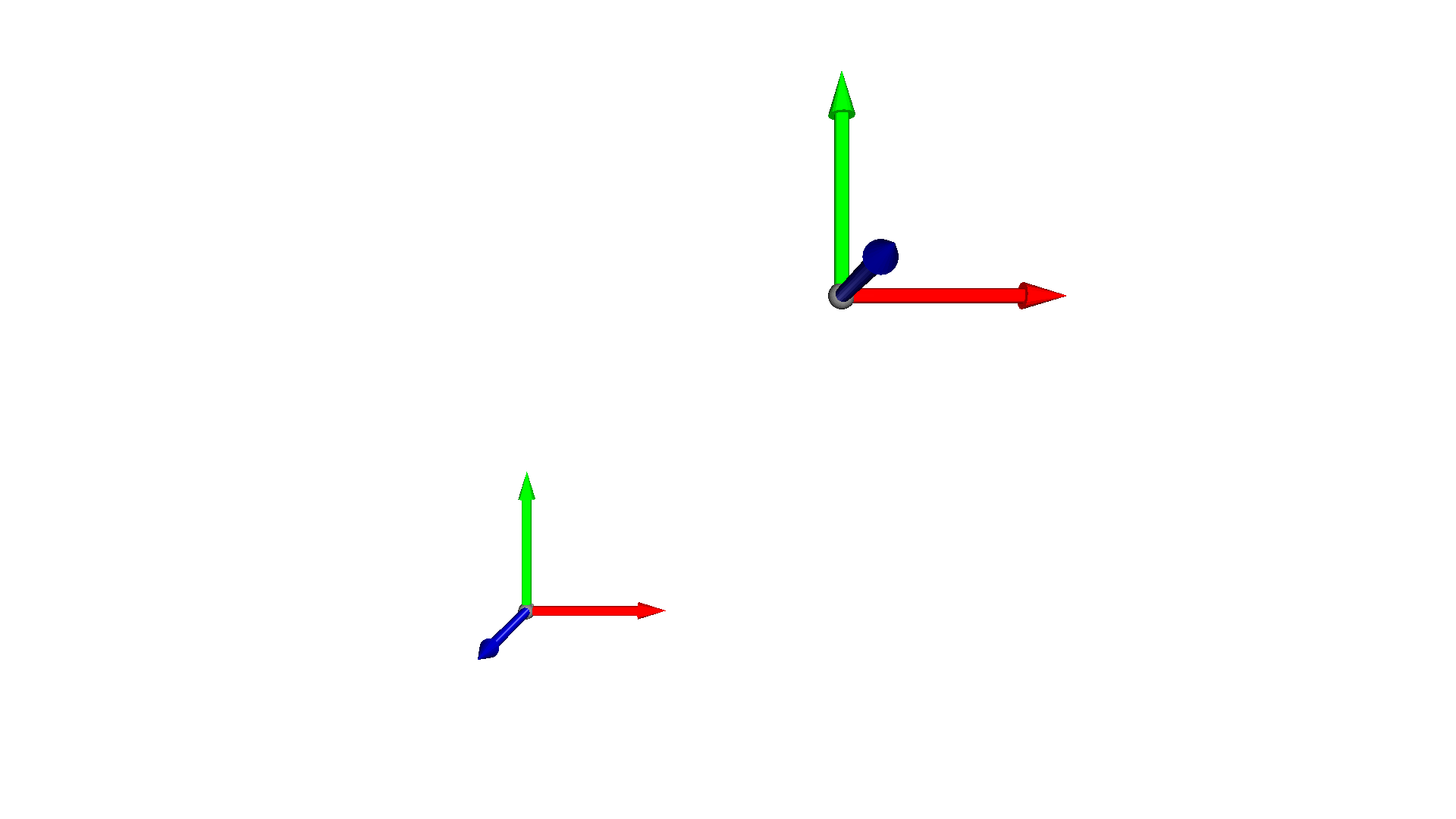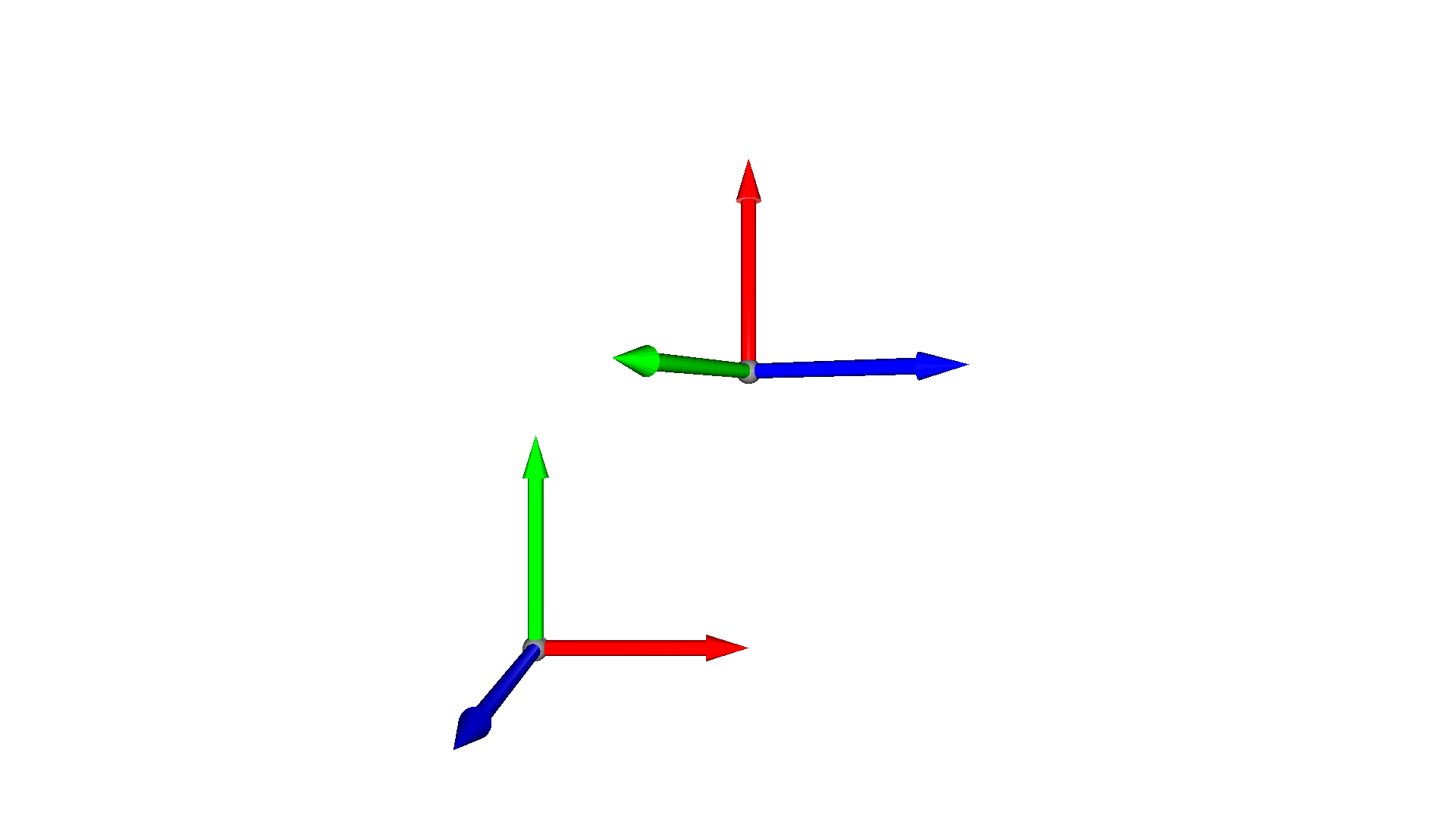# Transformation¶

The geometry types of Open3D have a number of transformation methods. In this tutorial we show how to use translate, rotate, scale, and transform.

## Translate¶

The first transformation method we want to look at is translate. The translate method takes a single 3D vector $$t$$ as input and translates all points/vertices of the geometry by this vector, $$v_t = v + t$$. The code below shows how the mesh is translated once in the x-directon and once in the y-direction.

:

mesh = o3d.geometry.TriangleMesh.create_coordinate_frame()
mesh_tx = copy.deepcopy(mesh).translate((1.3, 0, 0))
mesh_ty = copy.deepcopy(mesh).translate((0, 1.3, 0))
print(f'Center of mesh: {mesh.get_center()}')
print(f'Center of mesh tx: {mesh_tx.get_center()}')
print(f'Center of mesh ty: {mesh_ty.get_center()}')
o3d.visualization.draw_geometries([mesh, mesh_tx, mesh_ty])

Center of mesh: [0.05167549 0.05167549 0.05167549]
Center of mesh tx: [1.35167549 0.05167549 0.05167549]
Center of mesh ty: [0.05167549 1.35167549 0.05167549]Note:

The method get_center returns the mean of the TriangleMesh vertices. That means that for a coordinate frame created at the origin [0,0,0], get_center will return [0.05167549 0.05167549 0.05167549].

The method takes a second argument relative that is by default set to True. If set to False, the center of the geometry is translated directly to the position specified in the first argument.

:

mesh = o3d.geometry.TriangleMesh.create_coordinate_frame()
mesh_mv = copy.deepcopy(mesh).translate((2, 2, 2), relative=False)
print(f'Center of mesh: {mesh.get_center()}')
print(f'Center of translated mesh: {mesh_mv.get_center()}')
o3d.visualization.draw_geometries([mesh, mesh_mv])

Center of mesh: [0.05167549 0.05167549 0.05167549]
Center of translated mesh: [2. 2. 2.]## Rotation¶

The geometry types of Open3D can also be rotated with the method rotate. It takes as first argument a rotation matrix R. As rotations in 3D can be parametrized in a number of ways, Open3D provides convenience functions to convert from different parametrizations to rotation matrices:

• Convert from Euler angles with get_rotation_matrix_from_xyz (where xyz can also be of the form yzx, zxy, xzy, zyx, and yxz)

• Convert from Axis-angle representation with get_rotation_matrix_from_axis_angle

• Convert from Quaternions with get_rotation_matrix_from_quaternion

In the code below we rotate the mesh using Euler angles.

:

mesh = o3d.geometry.TriangleMesh.create_coordinate_frame()
mesh_r = copy.deepcopy(mesh)
R = mesh.get_rotation_matrix_from_xyz((np.pi / 2, 0, np.pi / 4))
mesh_r.rotate(R, center=(0, 0, 0))
o3d.visualization.draw_geometries([mesh, mesh_r])The function rotate has a second argument center that is by default set to True. This indicates that the object is first centered prior to applying the rotation and then moved back to its previous center. If this argument is set to False, then the rotation will be applied directly, such that the whole geometry is rotated around the coordinate center. This implies that the mesh center can be changed after the rotation.

:

mesh = o3d.geometry.TriangleMesh.create_coordinate_frame()
mesh_r = copy.deepcopy(mesh).translate((2, 0, 0))
mesh_r.rotate(mesh.get_rotation_matrix_from_xyz((np.pi / 2, 0, np.pi / 4)),
center=(0, 0, 0))
o3d.visualization.draw_geometries([mesh, mesh_r])## Scale¶

Vertices and points of Open3D geometry types can also be scaled using scale, $$v_s = s \cdot v$$.

:

mesh = o3d.geometry.TriangleMesh.create_coordinate_frame()
mesh_s = copy.deepcopy(mesh).translate((2, 0, 0))
mesh_s.scale(0.5, center=mesh_s.get_center())
o3d.visualization.draw_geometries([mesh, mesh_s])The scale method also has a second argument center that is set to True by default. If it is set to False, then the object is not centered prior to scaling such that the center of the object can move due to the scaling operation.

:

mesh = o3d.geometry.TriangleMesh.create_coordinate_frame()
mesh_s = copy.deepcopy(mesh).translate((2, 1, 0))
mesh_s.scale(0.5, center=(0, 0, 0))
o3d.visualization.draw_geometries([mesh, mesh_s])## General transformation¶

Open3D also supports a general transformation defined by a $$4\times4$$ homogeneous transformation matrix using the method transform.

:

mesh = o3d.geometry.TriangleMesh.create_coordinate_frame()
T = np.eye(4)
T[:3, :3] = mesh.get_rotation_matrix_from_xyz((0, np.pi / 3, np.pi / 2))
T[0, 3] = 1
T[1, 3] = 1.3
print(T)
mesh_t = copy.deepcopy(mesh).transform(T)
o3d.visualization.draw_geometries([mesh, mesh_t])

[[ 3.06161700e-17 -5.00000000e-01  8.66025404e-01  1.00000000e+00]
[ 1.00000000e+00  6.12323400e-17  0.00000000e+00  1.30000000e+00]
[-5.30287619e-17  8.66025404e-01  5.00000000e-01  0.00000000e+00]
[ 0.00000000e+00  0.00000000e+00  0.00000000e+00  1.00000000e+00]]